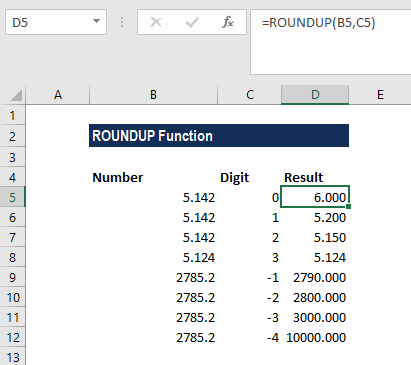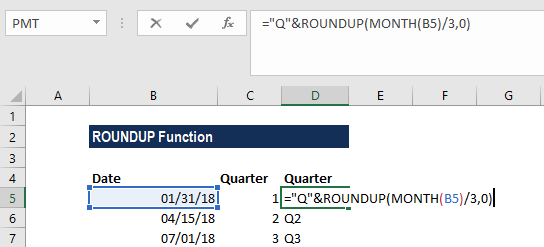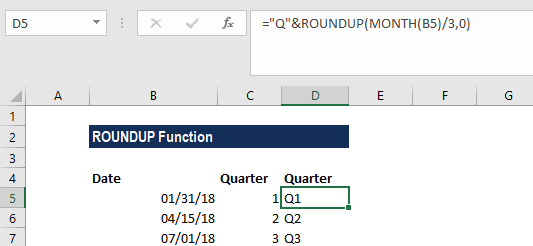# ROUNDUP Function

Rounds up a given number away from zero

## What is the ROUNDUP Function?

The ROUNDUP Function is categorized under Excel Math and Trigonometry functions. The function will round up a number away from zero.

As a financial analyst, the ROUNDUP function is useful as it helps round a number and eliminates the least significant digits, simplifying the notation but keeping close to the original value.

### Formula

=ROUNDUP(number,num_digits)

The ROUNDUP function uses the following arguments:

1. Number (required argument) – This is a real number that we wish to round up.
2. Num_digits (required argument) – This is the number of digits to which we want to round the number.

Now, if the num_digits argument is:

1. A positive value, it specifies the number of digits to the right of the decimal point.
2. Equal to zero, it specifies rounding to the nearest integer.
3. A negative value, it specifies the number of digits to the left of the decimal point.

### How to use the ROUNDUP Function in Excel?

To understand the uses of the ROUNDUP function, let’s consider a few examples:

#### Example 1

Suppose we are given the following data:

NumberDigitsRemarks
5.1420Round up to the nearest whole number
5.1421Round up to 1 decimal place
5.1422Round up to 2 decimal places
2785.23Round up to 3 decimal places
2785.2-1Round up to the nearest 10
2785.2-2Round up to the nearest 100
2785.2-3Round up to the nearest 1000
2785.2-4Round up to the nearest 10000

The results we get in Excel are shown below:#### Example 2

Suppose we are given the following data:

DateQuarter
01/31/181
04/15/182
07/01/183

If we wish to calculate the quarter (i.e., 1, 2, 3, 4) from a date, we can use this function together with the MONTH function. The formula to be used is:The formula above extracts the month as a number between 1-12, then divides the number by 3. The result is then rounded up to the nearest whole number using the ROUNDUP function. As we concatenated Q with the formula, we get the result below:### Things to remember about the ROUNDUP Function

1. ROUNDUP behaves like ROUND, except that it always rounds a number up.
2. ROUNDUP can round either to the left or right of the decimal point.
3. Unlike standard rounding, where numbers less than 5 are rounded up, the function always rounds numbers 1-9 up.

Thanks for reading CFI’s guide to the Excel ROUNDUP function. By taking the time to learn and master these Excel functions, you’ll significantly speed up your financial analysis. To learn more, check out these additional CFI resources:

• Excel Functions for Finance